Report a Problem
Suggestions

# The Mean Longitude Of Planets In Our Solar System

Last updated: Thursday, June 01, 2023

Mean longitude, in the context of planets, refers to the average angular position of a planet along its orbit around the Sun. It is a calculated value that provides information about the planet's location in its orbital path at a given time.

The mean longitude is measured in degrees and represents the angle between the planet's position and a reference point, such as the vernal equinox or a defined starting point in the planet's orbit. It serves as a way to track and predict the planet's position along its elliptical path.

The mean longitude takes into account the planet's orbital motion, which is not uniform due to gravitational interactions with other celestial bodies and the shape of the planet's orbit. It is an averaged value that smooths out these irregularities and provides a consistent measure of the planet's orbital position.

To calculate the mean longitude, astronomers use mathematical models that take into account the planet's orbital parameters, such as its semi-major axis, eccentricity, and inclination. These models incorporate Kepler's laws of planetary motion and consider the gravitational influences of other celestial bodies to estimate the planet's average position.

The Mean Longitude Of Planets In Our Solar System
Name Degrees Ratio To Earth Value
Moon0 0
Jupiter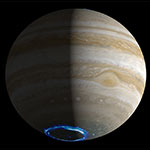34.40438 0.3425
Saturn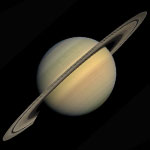49.94432 0.4971
Earth100.46435 1
Venus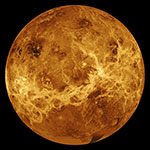181.97973 1.8114
Pluto238.92881 2.3782
Mercury252.25084 2.5108
Neptune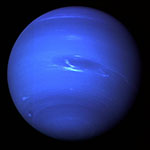304.88003 3.0347
Uranus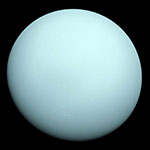313.23218 3.1178
Mars355.45332 3.5381
Source: NASA